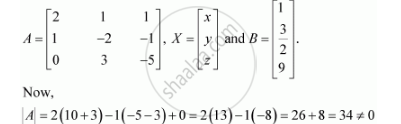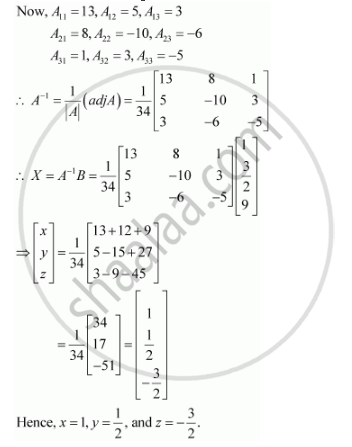Share

# Solve System of Linear Equations, Using Matrix Method. 2x + Y + Z = 1 X – 2y – Z = 3/2 3y – 5z = 9 - CBSE (Science) Class 12 - Mathematics

ConceptApplications of Determinants and Matrices

#### Question

Solve system of linear equations, using matrix method.

2x + y + z = 1

x – 2y – z = 3/2

3y – 5z = 9

#### Solution

The given system of equations can be written in the form of AX = B, whereThus, A is non-singular. Therefore, its inverse exists.Is there an error in this question or solution?

#### Video TutorialsVIEW ALL 

Solution Solve System of Linear Equations, Using Matrix Method. 2x + Y + Z = 1 X – 2y – Z = 3/2 3y – 5z = 9 Concept: Applications of Determinants and Matrices.
S